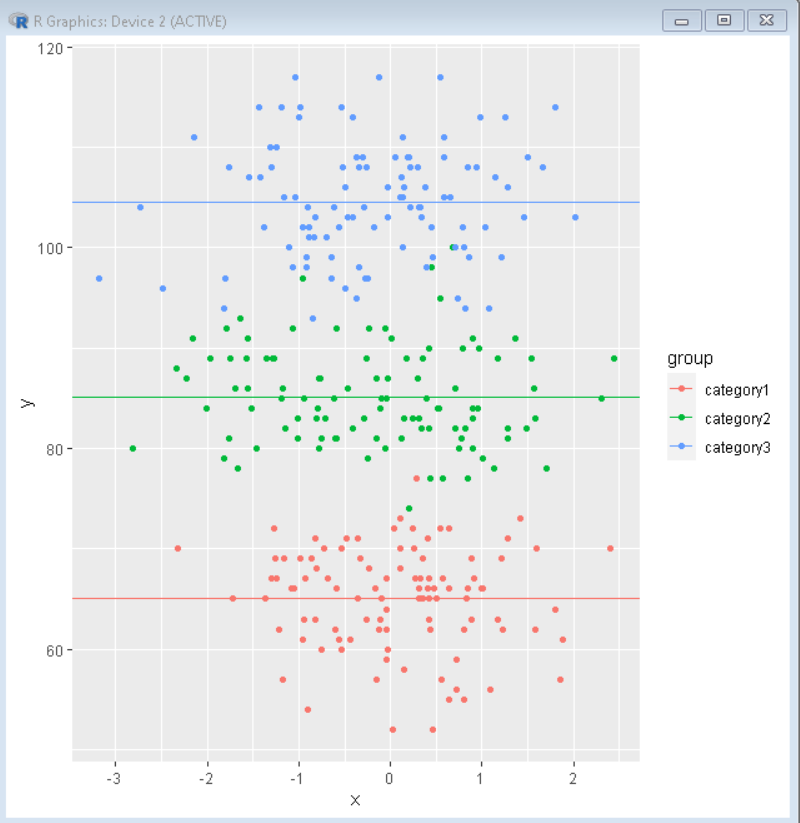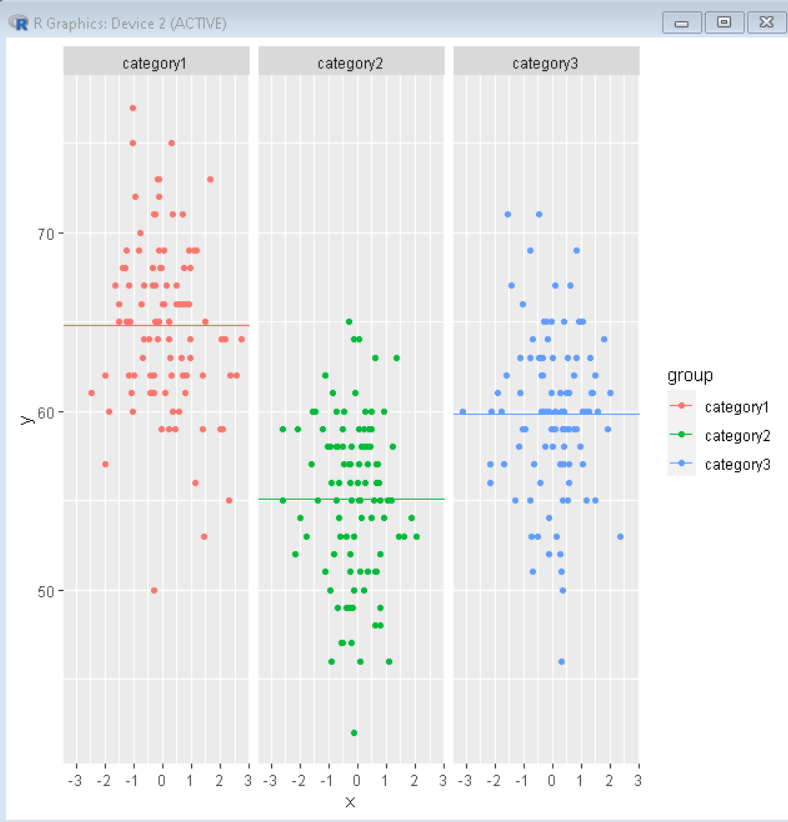# Add line for average per group using ggplot2 package in R

• Last Updated : 03 Dec, 2021

In this article, we will discuss how to add a line for average per group in a scatter plot in the R Programming Language.

In the R Language, we can do so by creating a mean vector by using the group_by() and summarise() function. Then we can use that mean vector along with the geom_hline() function of the ggplot2 package to create a line by the mean point colored by the group.

To create a mean vector from the data frame,

Syntax:

```mean <- df %>%
group_by( <categorical-variable> ) %>%
summarise( mean_val = mean( <quantitative-variable> )```

Arguments:

• df: determines the data frame to be used.
• <categorical-variable>: determines the variable that is used to divide data into groups.
• <quantitative-variable>: determines the variable whose mean is to be found.

This expression creates a vector with two columns i.e. <categorical-variable> and the mean that stores mean by category. Now, we will use this mean vector with the geom_hline() function to add a horizontal line at the mean/average of data colored by categorical variable.

Syntax:

`plot + geom_hline( mean_df, aes( yintercept, col )`

Arguments:

• mean_df: determines the data frame that contains mean information.
• yintercept: determines the variable mean column in dataframe.
• col: determines the categorical variable by which line has to be colored.

Example 1:

Here in this example, we have created a scatter plot colored by a categorical variable. Then we have added a line colored by the same variable that goes through the mean of that category of data.

## R

 `# load library tidyverse` `library``(tidyverse)`   `# create dataframe` `df <- ``data.frame``(` `    ``group=``factor``(``rep``(``c``(``"category1"``, ``"category2"``,``"category3"``),` `                        ``each=100)),` `    ``y=``round``(``c``(``rnorm``(100, mean=65, sd=5),` `                  ``rnorm``(100, mean=85, sd=5),` `                 ``rnorm``(100, mean=105, sd=5))),` `    ``x=``rnorm``(300))`   `# create mean by group` `mean <- df%>% ``group_by``(group)%>%``summarise``(mean_val=``mean``(y))`   `# create ggplot scatter plot` `# add horizontal line overlay at mean using geom_hline()` `ggplot``(data = df, ``aes``(x= x, y=y)) + ` `geom_point``(``aes``(colour = group)) + ` `geom_hline``(data= mean, ``aes``(yintercept = mean_val,col=group))`

Output:Example 2:

In this example, we have created a scatter plot colored by a categorical variable. Then we have added a line colored by the same variable that goes through the mean of that category of data. We have also added a facet_grid() to convert this plot into a facet plot to better visualize the data through a categorical variable.

## R

 `# load library tidyverse` `library``(tidyverse)`   `# create dataframe` `df <- ``data.frame``(` `    ``group=``factor``(``rep``(``c``(``"category1"``, ``"category2"``,``"category3"``),` `                        ``each=100)),` `    ``y=``round``(``c``(``rnorm``(100, mean=65, sd=5),` `                  ``rnorm``(100, mean=55, sd=5),` `                 ``rnorm``(100, mean=60, sd=5))),` `    ``x=``rnorm``(300))`   `# create mean by group` `mean <- df%>% ``group_by``(group)%>%``summarise``(mean_val=``mean``(y))`   `# create ggplot scatter plot` `# add horizontal line overlay at mean using geom_hline()` `# divide plot in facet using function facet_grid()` `ggplot``(data = df, ``aes``(x= x, y=y)) + ` `geom_point``(``aes``(colour = group)) + ` `geom_hline``(data= mean, ``aes``(yintercept = mean_val,col=group))+` `facet_grid``(~group)`

Output:My Personal Notes arrow_drop_up
Recommended Articles
Page :